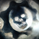14734 vues
14734
This is Version - 2 of the Momentum Oscillator, Like i said on the prior version, This version has an Indicator for Trends.

Marks only Overbought and Oversold Based on the TREND.

The Trend dots are calculated exactly like the Slingshot system trend.

```// Created by UCSgears
// Version 2

study(title="UCS_Momentum Oscillator", shorttitle="UCS_Osc")

// Stochastic Based Relative Oversold and Overbought

//Input
// Short Stochastic Input
length1 = input(8, minval=1, title = "Short %K")
sma1 = input(3, minval=1, title="Smooth %K1")

// Base Stochastic Input
length3 = input(100, minval=1, title = "Base %K")
sma3 = input(10, minval=1, title="Smooth %K3")

// Stochastic Code

k1 = (sma(stoch(close, high, low, length1), sma1)-50)
k3 = (sma(stoch(close, high, low, length3), sma3)-50)

//Histogram 1 Calc and Plot

hist1 = (k1-k3)
plot_color1 = hist1 > hist1 and hist1 > 0 ? green : hist1 < hist1 and hist1 > 0 ? blue : hist1 < hist1 and hist1 <= 0 ? red : hist1 > hist1 and hist1 <= 0 ? orange : white
plot(hist1, color=plot_color1, style=histogram, linewidth=4, title="Diff")

//Horizontal Lines

h1=hline(80, "Extreme Up", red, solid, 3)
h2=hline(-80, "Extreme Down", green, solid, 3)
h3=hline(55, "OverBought", red, dashed, 1)
h4=hline(-55, "OverSold", green, dashed, 1)
fill(h2,h4, green, 80, "OverSold Zone")
fill(h1,h3, red, 80, "OverBought Zone")

//Code for Trend Based Zero Line
ma1 = ema(close, 13)
ma2 = ema(close, 21)
ma3 = ema(close, 34)

ma = ema(close, 89)
range =  tr
rangema = ema(range, 89)
upper = ma + rangema * 0.5
lower = ma - rangema * 0.5
tr_up = ma1 > upper and ma2 > upper and ma3 > upper
tr_down = ma1 < lower and ma2 < lower and ma3 < lower
scolor = tr_up ? green : tr_down ? red : blue
plot(0, color=scolor, style=circles, linewidth=3)```Hi , may I know your thought flow on the choice for the short & base stochastic input (i.e. 8/3, 100/10)?
I combine this MO with CM_UCS_Modified SlingShot and it works great in identifying real trend when
MO reach the red / green zone and find that up / down trend usually follows subsequently.